## Indicators

•Cited by SciELO
•Access statistics

•Cited by Google
•Similars in SciELO
•Similars in Google

## Print version ISSN 0121-7488

### Ciencia en Desarrollo vol.9 no.1 Tunja Jan./June 2018

Artículos

Analysis of the diffraction efficiency in transverse configuration in sillenite crystals Bi12TiO 20

Análisis de la eficiencia de difracción en configuración transversal en cristales silenitas Bi12TiO20

Astrid Lorena Villamizar Amadoa  , Néstor Alonso Arias Hernándezb  , Martha Lucía Molina Pradob  , Myrian Tebaldic  *

a Grupo de Óptica Moderna, Departamento de Física y Geología, Universidad de Pamplona.

b Grupo de Óptica Moderna, Departamento de Física y Geología, Universidad de Pamplona.

c Centro de Investigaciones Ópticas (CONICET La Plata, CIC) and UIDET OPTIMO, Facultad Ingeniería, Universidad Nacional de La Plata, Argentina.

Abstract

A theoretical study on sillenite crystal where it obtained an analytics expression for diffraction efficiency in the on-Bragg regime in transverse configuration (K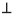< 001 >) is realized. It is considered non-mobile transmission gratings. An analysis of diffraction efficiency considering the non-uniformity of gratings, material properties, external parameters the applied field or the intensity of beam input or polarization or the optical activity of the crystal beetwen others is realized.

Key words: Photorefractive crystals; diffraction efficiency

Resumen

Se realiza un estudio teórico en cristales silenitas, donde se obtiene una expresión analítica para la eficiencia de difracción en el régimen de Bragg, en la configuración transversal (K< 001 >). Se considera redes no móviles de transmisión. Se realiza un análisis de la eficiencia de difracción considerando la no-uniformidad de las redes, propiedades del material, parámetros externos como campo aplicado o la intensidad de los haces de entrada o la polarización o la actividad óptica del cristal, etc.

Palabras-clave: Cristales fotorrefractivos; eficiencia de difracción

1. INTRODUCCION

Sillenite crystals are cubic, photorefractive and electro-optical crystals, and are studied for their different applications, such as non-linear signals, optical interconnections and optical space. These material has electro-optical coupling, birefringence and optical activity. The photorefractive effect is the resposible the of refractive index change in the material for the space charge and the applied field. In this work we considered the polarization beams and non-moving transmission gratings. We calculated the diffraction efficiency fot the transverse configuration in Bragg regime. We considered the variation of fringe period. One crystals orientation is considered, with the grating vector KG is perpendicular to the crystallographic axis <001>

2. SET COUPLE WAVE EQUATION

The BTO is a material with electrooptic coupling and optical activity, the wave equation that governs light propagation is:

Where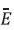is the total light field,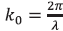is the magnitude of the optical wave vector,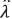is the wavelength and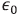is the free space permittivity.

For a birefringence medium with optical activity and electrooptic coupling, this constitutive relation can be expressed in the form:

Where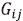is the optical activity antisymemetric tensor,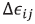is the change in the permittivity tensor induced by the linear Pockels electro optic effect and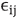is the symmetric optical permittivity tensor in the absence of optical activity and electro-optic coupling.

For the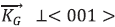orientation, the x axis is parallel to the  crystallographic face, as show in Fig. 1. The transformation matrix that converts from the crystallographic system to the light propagation system is:

For this orientation, the permittivity tensor exclusive of optical activity components, is:

where ,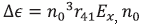is the index of refraction,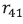is electrooptic coefficient and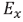is the electric field inside of crystal. This field consists of two components:

Is the applied electric field and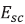is the space charge field, which depend of the diffusion and saturation fields. In the steady-state saturation limit, the space charge fieldis given by

In Eq. (6) E0 is the applied electric field of eq. (5), Ed is the diffusion field and Eq is an upper limit on the space charge field corresponding in a single trap model to saturation of available acceptor site (assuming monopolar charge transport in which electrons are the only mobile carriers). The diffusion field is defined by

where kB is Boltzmann's constant, T is the temperature and e is the electronic charge, the upper limit on the space charge field Eq is defined

where NA is the trap (acceptor) number density,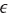is the low-frequency dielectric constant and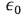is the permittivity of free space.

The incident probe beam R is assumed to be well collimated with a small propagation angle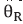with respect to the z axis (Fig. 2). The signal beam will also be well collimated (assuming a single grating frequency) with a small propagation angle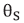with respect to the z axis. Propagation angles obtained by rotating the propagation direction away from the z axis toward the +x direction (i.e., a clockwise rotation in Fig. 2) are defined to be negative. Thus, in Fig. 2, the probe beam R has a positive propagation angle while the diffracted signal beam S has a negative angle. The total light field of the two beams can be written as:

The polarization states of the incident probe beam R and of the diffracted signal field S are two orthogonal linear polarization states which components is given by:

The set of coupled wave equations can be derived now that structure of the light field has been defined. The optical activity tensor can be added to the permittivity tensor, the constitutive relation for the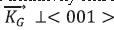> orientation is:

Where is a parameter defined by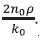.

Using the slowly varying envelope approximation derives the coupled wave equations. The resulting coupled wave equations are:

where is the optical rotatory power and for convenience in eqs.(13-16) we define birefringence parameters Co and Csc by

For the transverse configuration we obtained the diffraction efficiency n, defined by

where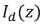is the intensity of the diffraction light,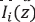the intensity of the incident light.

3. RESULT AND DISCUSSION

The theoretical analysis was realized using the Runge-Kutta method and its algorithm in the software Matlab.

In Fig. 3 we show the result for the dependence of the diffraction efficiency on the sample thickness for different applied field for a BTO grating using red light (632 nm) for reading. For this case,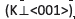, m =0.9 and F=179mm-1; the largest value of the diffraction efficiency occurs for an applied field of 10 kV/cm (see Table 1). We can see that the smallest of diffraction efficiency occurs for the smallest value of the applied field 1 kV/cm.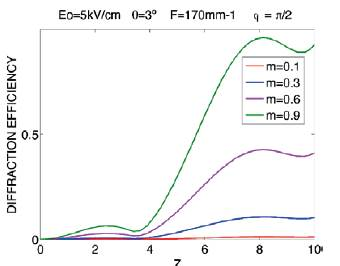Figura 3 BTO crystal diffraction efficiency in terms of the crystal thicknesses using red read-out light and different applied electric fields Ea= 1kV/cm, 5kV/cm, 10kV/cm,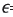=47, m=0.9, F=179mm-1

Table 1 Parameters for BTO[11,16,17,19] takes for our calculations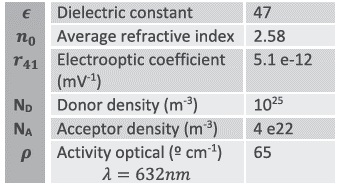In Fig. 4 we show the result for the dependence of the diffraction efficiency on the sample thickness for a BTO grating using red light (632 nm) for reading for different grating. For this case,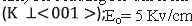; m = 0.9, m = 0.6, m = 0.3 and m = 0.1. The largest value of the diffraction efficiency occurs for modulation of 0.9.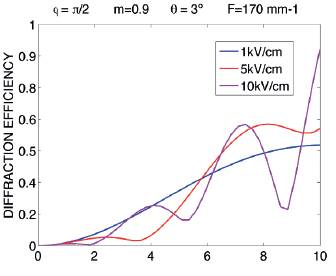Figura 4 BTO crystal diffraction efficiency in terms of the crystal thicknesses using red read-out light and different modulation m = 0.9,m = 0.6, m = 0.3 and m = 0.1 for E0 =5kV/cm

In Fig. 5 we show the result for the dependence of the diffraction efficiency on sample thickness for a BTO grating using red-out light (632 nm) for reading for different grating. For this case: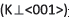; Eo=5 Kv/cm, m = 0.9;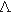= 5µm, 10 (µm and 20(µm. In this case, the largest value of the diffraction efficiency occurs for a period of 20(µm. We can see that the smallest of diffraction efficiency occurs for the smallest value of period 5(µm, the largest value of the diffraction efficiency occurs for a period of 20µm.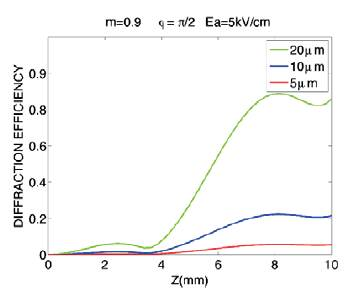Figura 5 BTO crystal diffraction efficiency in terms of the crystal thicknesses using red read-out light for A=5|im, A=10^m, A=20^m with E0=5kV/cm and m=0.9

4. CONCLUSION

We studied the diffraction efficiency in non-moving transmission gratings, in Bragg regime in transversal configuration in BTO crystal. A set of coupled-wave equations has been derived for transverse holographic orientation in crystal BTO of sillenite family. These equations allow an analysis to be made of the polarization properties of light diffraction including the effects of concomitant optical rotatory power. In the date analytic we used red light for the reading. We considered the birefringence, coupling electro-optic, optical activity, fringe period, different fields applied, different modulations and the polarization beams.

The results show that for a modulation of 0.9 the diffraction efficiency is greater that for a modulation of m=0.1. Also we can see that for the field applied of 1kv/cm correspond to the small diffraction efficiency while that for the field applied of 5kv/cm the diffraction efficiency is more stable. Too, for the fringe period of 5(µm the diffraction efficiency is smallest that for a period of 20 (µm. In conclusion, the thickness of sample in where occur the diffraction efficiency largest is in a crystal of 8mm.

REFERENCES

 P. Yeh, "Introduction to Photorefractive Nonlinear Optics ", Wiley, New York, 1993. [ Links ]

 L.F. Magaña, I. Casar, J.G. Murillo, Beam energy exchange in sillenite crystals (Bi-i2SiO20 and Bi¡2TiO2o), considering the variation of light modulation along sample thickness in a strong non-linear regime, Opt. Mater. 30 (2008) 979-986. [ Links ]

 Marrachi, A., Johnson, R.V, Tanguay, Jr.A.R "Polarization properties of photorefractive diffraction in electrooptic and optically active sillenite crystals (Bragg Regime), J. Opt. Som.A.B, 1986 [ Links ]

 Gonzales, G., Zuñiga, A. "Optimization of the diffraction efficiency in nonuniform gratings in sillenite crystals considering the variation of fringe period, optical activity and polarization angles in a strong non-linear regime" , Revista Mexicana de Física, 2009, 55(1):25-31, 2009. VII, 3, 42, 43, 50 [ Links ]

 Molina Prado M.L., et al. "Medida de la eficiencia de difracción fotorrefractiva como función del vector de onda de la red en un cristal BTO ", Bistua, 9 (2), 43-47(2011). [ Links ]

 Molina M.L. et al, "Eficiencia de difracción del registro de speckle modulados generados a partir de superficies reflecto-difusoras ", BISTUA, Vol 10 (2), 2012. [ Links ]

Received: August 27, 2017; Accepted: October 10, 2017This is an open-access article distributed under the terms of the Creative Commons Attribution License